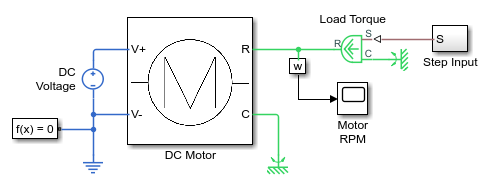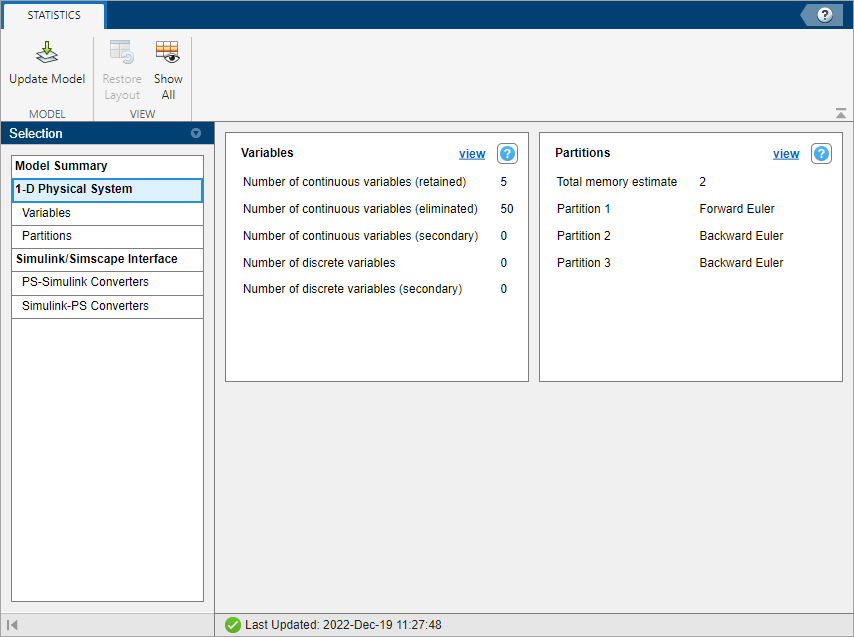## Partitioning Solver Statistics

If your model uses the local Partitioning Solver, the Statistics Viewer tool provides additional details about the partitions.

• Solver Type — Type of solver for a given partition.

• Equation Type — Type of equations for a given partition. Possible types are linear time-invariant, switched linear, and linear time-varying.

• Variables — Number of scalar variables in the partition. To learn more, visit Access Block Variables Using Statistics Viewer.

• Equations — Number of scalar equations in the partition.

• Modes — Number of linear time-invariant or switched linear modes in the partition. Every `if` and `elseif` statement in Simscape™ source code corresponds to a mode. When the solver uses implicit integration in a partition, additional modes tend to result in additional necessary iterations.

• Configurations — Total number of different systems of linear equations that need to be solved when simulating the partition. In the linear time-invariant or switched linear cases, this is 2n, where n is the number of modes in the partition. To accelerate computation, the solver caches decompositions of some systems for each set of modes as defined by the Partition storage method parameter in the Solver Configuration block. If this number exceeds the greatest possible supported unsigned integer value, the statistic displays `Overflow`.

• Memory Estimate — Estimate for memory use, in kB, for this partition when using the exhaustive partition storage method.

### Estimate the Memory Budget for Exhaustive Partitioning Storage

This example shows how you can use the Statistics Viewer to estimate the memory budget needed for simulating a model that uses the Partitioning solver.

1. Open the Permanent Magnet DC Motor example model.2. Double-click the Solver Configuration block, select the Use local solver check box, and then set the Solver type to `Partitioning`.

3. To view model statistics, in the model window, on the Debug tab, click Simscape > Statistics Viewer. Click the to populate the viewer with data.

4. Select 1-D Physical System.The Total memory estimate statistic indicates that the estimate for memory usage for this model is 2 kB. When you use the exhaustive partition storage method, the default memory budget allocated for partition storage is 1024 kB. The default memory budget value is sufficient. To see the memory use estimate for a given partition, select Partitions.